QRコード(所蔵情報)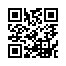### Recent advances in differential equations and mathematical physics : UAB International Conference on Differential Equations and Mathematical Physics, March 29-April 2, 2005, University of Alabama at Birmingham

フォーマット:

Nikolai Chernov ... [et al.], editors

Providence, R.I. : American Mathematical Society, c2006

xvii, 333 p. : ill. ; 26 cm

シリーズ名:
Contemporary mathematics ; 412 <BA00009927>

BA78031425
ISBN:
9780821838402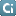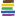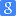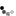### 類似資料:

1

2

3

4

5

6

7

8

9

10

11

12

 1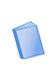図書 Advances in differential equations and mathematical physics : UAB International Conference, Differential Equations and Mathematical Physics, March 26-30, 2002, … International Conference on Differential Equations and Mathematical Physics, Karpeshina, Yulia E., 1956- American Mathematical Society 7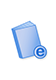電子ブック Advances in differential equations and mathematical physics Karpeshina, Yulia E. American Mathematical Society 2図書 Advances in differential equations and mathematical physics : 1997 Georgia Tech-UAB International Conference on Differential Equations and Mathematical Physics, … International Conference on Differential Equations and Mathematical Physics, Carlen, Eric, 1957-, Harrell, Evans M., … American Mathematical Society 8図書 Differential equations and mathematical physics : proceedings of the international conference held at the University of Alabama at Birmingham, March 15-21, 1990 International Conference on Differential Equations and Mathematical Physics, Bennewitz, Christer, 1943- Academic Press 3電子ブック Recent advances in differential equations and mathematical physics Chernov, Nikolai, Karpeshina, Yulia American Mathematical Society 9電子ブック Differential Equations and Mathematical Physics Ian W. Knowles Springer Berlin Heidelberg 4図書 Differential equations : proceedings of the conference held at the University of Alabama in Birmingham, Birmingham, Alabama, U.S.A., 21-26 March, 1983 Lewis, Roger T., 1942-, International Conference on Differential Equations, Knowles, Ian W., 1946- North-Holland, Sole distributors for the U.S.A. and Canada, Elsevier Science Pub. Co. 10図書 Partial differential operators and mathematical physics : International Conference in Holzhau, Germany, July 3-9, 1994 Demuth, Michael, Schulze, Bert-Wolfgang, International Conference on "Partial Differential Equations" Birkhauser 5図書 Differential equations and mathematical physics : proceedings of an international conference held at the University of Alabama in Birmingham, March 16-20, 1999 Weikard, Rudi, Weinstein, Gilbert, International Conference on Differential Equations and Mathematical Physics American Mathematical Society, International Press 11図書 Differential equations and applications : proceedings of the International Conference on Theory and Applications of Differential Equations, Columbus, Ohio, 1988 International Conference on Theory and Applications of Differential Equations, Aftabizadeh, A. R. (A. Reza) Ohio University Press 6図書 Differential equations and mathematical physics : proceedings of the conference sponsored by University of Alabama at Birmingham and Georgia Institute of … Knowles, Ian International Press 12図書 Differential equations and control theory : proceedings of the International Conference on Differential Equations and Control Theory, Wuhan, People's Republic … International Conference on Differential Equations and Control Theory, Teng, Tsung-chʿi M. Dekker